General 4x4 Magic Squares with Entries from Symmetric Sets
© H.B. Meyer         for classical 4x4 magic squares see here         zur Seite in deutscher Sprache         magic squares and cubes

 15 29 1 43 31 27 19 11 25 9 33 21 17 23 35 13

is a general 4x4 magic square:
- the 16 entries are different natural numbers,
- the 4 rows, the 4 columns and the 2 diagonals sum up to the same result (=88).

The set T={1,9,11,13,15,17,19,21,23,25,27,29,31,33,35,43} of entries is a symmetric set, that means: for any x in T the number N+a-x is also an element of T; here N=43 is the greatest element of T and a=1 the smallest.

Purpose of this website   General 4x4 magic squares with entries from symmetric sets (GMS) are studied:
Which and how many GMS do exist and what structure do they and their entry sets have.

Observation 1   It is sufficient to deal only with GMS, which have the number 1 as an entry: If the smallest entry is a, then subtraction of a-1 from all entries leads to a GMS with entry 1.
Every classical 4x4 magic square is a GMS.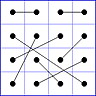Observation 2   For any general 4x4 magic square with entries from a symmetric set, containing 1, with greatest element N, the magic sum ist 2N+2. There are 8 pairs of entries with sum N+1. When the two entries of each such pair in the square are connected by a line, then the connection figure (CF) of the square is generated (here the connection figure of the above example GMS is shown). Any CF can be written as horizontal string with 16 entries, f.i. write (1122 3456 5768 4873) for the shown CF. Read the string as 4x4 matrix and connect equal entries by a line.

Observation 3   Let M be a GMS. If every entry x of M is replaced by N+1-x (N: greatest entry), then one gets another GMS with the same connection figure as M, it is called the complement of M. The entries of M in the green marked regions add up to 2(N+1):
 a b c d e f g h i j k l m n o p
 a b c d e f g h i j k l m n o p
 a b c d e f g h i j k l m n o p
 a b c d e f g h i j k l m n o p

Observation 4   If 01 represents a GMS then each of the 31 squares 02-32 represents a GMS, too:
01
 a b c d e f g h i j k l m n o p
02
 d h l p c g k o b f j n a e i m
03
 p o n m l k j i h g f e d c b a
04
 m i e a n j f b o k g c p l h d
05
 m n o p i j k l e f g h a b c d
06
 d c b a h g f e l k j i p o n m
07
 a e i m b f j n c g k o d h l p
08
 p l h d o k g c n j f b m i e a
09
 a c b d i k j l e g f h m o n p
10
 d l h p b j f n c k g o a i e m
11
 p n o m h f g e l j k i d b c a
12
 m e i a o g k c n f j b p h l d
13
 m o n p e g f h i k j l a c b d
14
 d b c a l j k i h f g e p n o m
15
 a i e m c k g o b j f n d l h p
16
 p h l d n f j b o g k c m e i a
17
 k i l j c a d b o m p n g e h f
18
 j b n f l d p h i a m e k c o g
19
 f h e g n p m o b d a c j l i k
20
 g o c k e m a i h p d l f n b j
21
 g e h f o m p n c a d b k i l j
22
 j l i k b d a c n p m o f h e g
23
 k c o g i a m e l d p h j b n f
24
 f n b j h p d l e m a i g o c k
25
 f e h g b a d c n m p o j i l k
26
 g c o k h d p l e a m i f b n j
27
 k l i j o p m n c d a b g h e f
28
 j n b f i m a e l p d h k o c g
29
 j i l k n m p o b a d c f e h g
30
 g h e f c d a b o p m n k l i j
31
 f b n j e a m i h d p l g c o k
32
 k o c g l p d h i m a e j n b f

These are the 32 transformations of GMS. 02, 03, and 04 represent rotations of 01, while 05 and 06 are reflections of 01 at a horizontal resp. vertical axis, and 07, 08 represent reflections of 01 at its diagonals.

Proposition 1  There exist 145 different connection figures for GMS.
This can be proved using linear algebra; a sketch of the proof can be found here, and a list with the 145 possible CF can be found here.

Definition  Call two connection figures strong equivalent, if they can be mapped onto each other by a rotation or reflection (a transformation 01-08 of Observation 4). Call two connection figures equivalent, when they can be mapped onto each other by any of the 32 transformations of Observation 4.

Example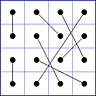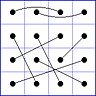The first and the second CF are strong equivalent (via transformation 02), and the first and third CF are equivalent (via transformation 14). In strings:(1122 3456 5768 4873) is strong equivalent to (1234 1526 7863 7458),(1122 3456 5768 4873) is equivalent to (1212 3456 5768 8347).

Proposition 2  The 145 CF for GMS can be divided into 34 equivalence classes, using strong equivalence, this means: there exist 34 different CF, when rotations or reflections are not regarded.
All 145 connection figures can be devided into 12 equivalence classes EC01,...,EC12, using only equivalence.
The 12 classes and the 34 connection figures are:

 EC01: (1122 3344 5566 7788), equivalent CF: 4[Dudeney IV,V] EC02: (1122 3443 5665 7788), equivalent CF: 8[Dudeney IX,VIII,VII,X]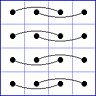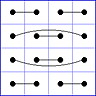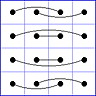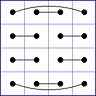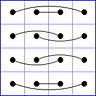EC03: (1122 3456 7788 4365), equivalent CF: 8[Dudeney XI,XII] EC04: (1122 3456 5678 8347), equivalent CF: 32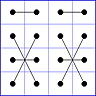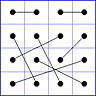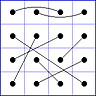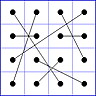EC05: (1122 3456 5768 4873), equivalent CF: 32 EC06: (1122 3443 7878 6543), equivalent CF: 16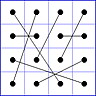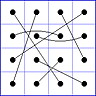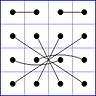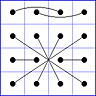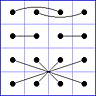EC07: (1122 3456 6783 8547), equivalent CF: 16 EC08: (1221 3443 5665 7887), equivalent CF: 2[Dudeney VI]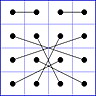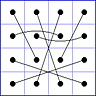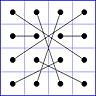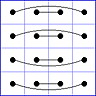EC09: (1234 5167 7385 2648), equivalent CF: 16 EC10: (1234 5678 8765 4321), equivalent CF: 1[Dudeney III]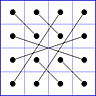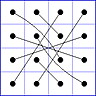EC11: (1234 2567 8653 4871), equivalent CF: 8 EC12: (1234 2143 5678 6587), equivalent CF: 2[Dudeney II,I]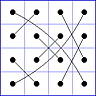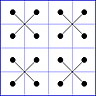Remark  Dudeneys class numbers I-XII for classical 4x4 magic squares (N=16) are notated in brackets.
The first (bordered) CF shown, together with the attached string, in each of the classes EC01-EC12 will be taken as representative R01-R12 of its class.

Observation 5  Suppose M is a GMS, then there exists a unique transformation 01-32 of Observation 4 which maps M onto a GMS with a<d,f,g,j,k,m,p (a is the smallest diagonal entry), d<m and b<c. Such 4x4 squares are called reduced. So every GMS can be mapped onto a unique reduced one.

Observation 6  Let T be a symmetric set of natural numbers (with 16 elements, containing the number 1), then there are two possibilities for counting the number of GMS belonging to a fixed CF with entries from T:
- count the number u of reduced GMS with entries from T, belonging to all equivalent CF,
- count the number v of all GMS with entries from T for the representative of the class of the fixed CF.
Obviously: 32u = vw, where w is the number (shown in Proposition 2) of members of the class of the fixed CF.

Proposition 3  For any symmetric set T (of 16 natural numbers, smallest element 1), one can write down the vector (u01|u02|...|u12), where uxy is the number of reduced GMS M with entries from T, where the CF of M belongs to the class ECxy. If the greatest number N of T is N<61 then there are exactly 154 possibilities for the vector (u01|u02|...|u12).
This was proved by computer experiment; a file of the 154 possible vectors can be found here.

Proposition 4  For 9 of the 12 classes the number of all GMS with entries from an arbitrary symmetric set for the representative Rxy allows only two values: 0 or a constant number vxy:

 class 01 02 03 04 05 06 07 08 09 10 11 12 vxy 384 v v 2 2 4 4 v 4 384 16 384

v means: variable, more than two possible values.

This was proved in Theorems 01-12 (see below).

Conjecture  There are no other possible vectors for 60<N than the 154 vectors (u01|u02|...|u12) from Proposition 4. This also means, that there are no other values for v02, v03, and v08 than those described in Theorem 02, Theorem 03 and Theorem 08.

12 Theorems  For each of the classes EC01-EC12 there are Theorems, dealing with the question: how many GMS for their representatives exist, and which structure these GMS and the sets of their entries can have. The 12 Theorems can be found here:
Theorem 01, Theorem 02, Theorem 03, Theorem 04, Theorem 05, Theorem 06, Theorem 07, Theorem 08, Theorem 09, Theorem 10, Theorem 11, Theorem 12.

Summary of selected statements

(S01)  The set T17={1,2,3,4,5,6,7,8,10,11,12,13,14,15,16,17} allows 260 reduced GMS, therefore 32*260=8320 GMS (compare with classical magic squares). That this number cannot be surpassed, is an old conjecture (see references below) and til today scientifically unproved, as well as the question whether there are other vectors than the named 154 ones .

There are symmetric sets, which allow as many GMS, as there are classical 4x4 magic squares, namely 7040. But the structure of these GMS can be rather different from the structure of classical magic squares (see Vectors154.pdf).
u  v
 p o n m l k j i h g f e d c b a a x z x w y w x y z y y b x z x y w w y x y z y c z x w y x x y w y z y d z x y w x y x w y y z e x w y x z z y y w x y f x y w x z y z y w y x g w y x z x y z y x y w h y w x z x y y z y x w i w x y z y y x z x w y j w y x y z y x z x y w k x y w y z y z x w y x l y x w y y z z x y w x m z y y w x y x w y x z n y z y w y x x y w x z o y z y x y w w y x z x p y y z y x w y w x z x
T17:

number of
4x4 magic
squares
with

u + v = 18

 w x y z
0 squares
32 squares
384 squares
608 squares
2336 squares

(S02)  The classes EC01, EC10, and EC12 are isomorphic, this means, that for any fixed symmetric set with 1, and any three CF, one in each class, there exist a one to one mapping from the set of all 384 GMS belonging to the CF of one class onto the set of all 384 GMS belonging to the CF of the other class. So any GMS, which has a CF from class EC01, EC10, or EC12 can be derived by 384 mappings from only the one classical 4x4 magic square

 1 16 4 13 6 11 7 10 15 2 14 3 12 5 9 8

(see Theorems 01, 10, and 12).
The entry sets of these GMS allow a "generalized binary decomposition" similar to the set {1,2,...,16}, where every element < 16 is a unique linear combination of 1,2,4,8 (see Theorem 1).

(S03)  The classes EC04 and EC05 are isomorphic, too. The sets of entries (containing 1) from GMS with CF of these classes are very special, their difference vector of entries always is t*(4,1,1,1,1,1,1,1,1,1,1,1,1,1,4) (see Theorems 4 and 5).

(S04)  There are several imbeddings of sets of GMS with connection figure Rxy (representative of ECxy) into the set of GMS with a CF, which is a representative of another class; in particular:
R03 --> R01 <--> R10 <--> R12 --> R08; R04 <--> R05 --> R02; R09 --> R02; R06,R07 --> R11 --> R02.
All these maps preserve the entry sets (see the corresponding theorems).

(S05)  The imbeddings of (S04) show: a symmetric set T allows a GMS if and only if T allows a GMS of CF R02 or R08.

(S06)  If a symmetric set T allows a GMS with entry 1, then T admits at least 128 GMS with entry 1; this follows from (S05) and Theorems 02 and 08.

(S07)  Let T be a symmetric set containing 1 and suppose, the greatest element N of T is odd. If the number of even elements x from T with x<N/2 is odd, then T allows no GMS. This follows from Theorems 02 and 08, observing the residues mod 2 of the elements of sets (*) and (**) in each Theorem.

References  Apparently the 34 connection figures were already mentionend 1986 in a japanese journal, see . The figures are published in , in an appendix of this book one can find an original discussion of the concept "fertility" of symmetric (and even non-symmetric) sets (fertility means the number of possible GMS for a fixed set); also see . The record of 260 reduced GMS for N=17, was conjectured in . The paper  deals among other things with the structure of GMS for various CF, and the 12 EC's are mentioned there.

 Gakuho Abe: Unsolved Problems on magic Squares, Discrete Mathematics Volume 127, Issues 1-3, 15 March 1994, Pages 3-13
 Y.V. Chebrakov, Analytical Formulae and Algorithms for Constructing Magic Squares from an arbirary Set of 16 Numbers, Smarandache Notations J. (1997) 8 (1-2-3)
 Harvey Heinz: Magic Squares Update 2009
 Lee Sallows: Geometric Magic Squares: A Challenging New Twist Using Colored Shapes Instead of Numbers, Dover Publications 2012

Last update: 2016/July/15